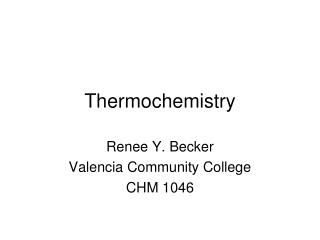DownloadDownload PresentationThermochemistry

# Thermochemistry

Download Presentation## Thermochemistry

- - - - - - - - - - - - - - - - - - - - - - - - - - - E N D - - - - - - - - - - - - - - - - - - - - - - - - - - -
##### Presentation Transcript

1. Thermochemistry Renee Y. Becker Valencia Community College CHM 1046

2. Enthalpies of Chemical Change:Often called heats of reaction (DH) units kJ Endothermic:Heat flows into the system from the surroundings and DH has a positive sign. Exothermic:Heat flows out of the system into the surroundings and DH has a negative sign.

3. Are the following reactions endothermic or exothermic? Favorable or unfavorable? C3H8(g) + 5 O2(g)  3 CO2(g) + 4 H2O(l) DH = –2219 kJ CH3Cl(g) + HCl(g)  CH4(g) + Cl2(g) DH = 98.3 kJ

4. Entropy • The measure of molecular disorder in a system is called the system’s entropy; this is denoted S. • Entropy has units of J/K (Joules per Kelvin). DS = Sfinal – Sinitial Positive value of DS indicates increased disorder. Negative value of DS indicates decreased disorder.

5. Entropy • Second Law of Thermodynamics: Reactions proceed in the direction that increases the entropy of the system plus surroundings. • A spontaneous process is one that proceeds on its own without any continuous external influence. • A nonspontaneous processtakes place only in the presence of a continuous external influence.

6. Predict whether DS is likely to be positive or negative for each of the following reactions. • a. 2 CO(g) + O2(g)  2 CO2(g) • b. 2 NaHCO3(s)  Na2CO3(s) + H2O(l) + CO2(g) • c. C2H4(g) + Br2(g)  CH2BrCH2Br(l) • d. 2 C2H6(g) + 7 O2(g)  4 CO2(g) + 6 H2O(g)

7. To decide whether a process is spontaneous, both enthalpy and entropy changes must be considered: Spontaneous process: Decrease in enthalpy (–DH). Increase in entropy (+DS). Nonspontaneous: Increase in enthalpy (+DH). Decrease in entropy (–DS).

8. Free Energy • Gibbs Free Energy Change (DG): Weighs the relative contributions of enthalpy and entropy to the overall spontaneity of a process. DG = DH – TDS • DG < 0 Process is spontaneous • DG = 0 Process is at equilibrium • DG > 0 Process is nonspontaneous

9. Which of the following reactions are spontaneous under standard conditions at 25°C? a. AgNO3(aq) + NaCl(aq)  AgCl(s) + NaNO3(aq) DG° = –55.7 kJ b. 2 C(s) + 2 H2(g)  C2H4(g)DG° = 68.1 kJ c. N2(g) + 3 H2(g)  2 NH3(g) DH° = –92 kJ; DS° = –199 J/K

10. Equilibrium (DG = 0): Equilibrium is the point where DG = DH – TDS = 0 • Estimate of the temperature at which a reaction becomes spontaneous (set DG =0) T = DH / DS

11. 2 NH3(g)  N2(g) + 3 H2(g) DH = 92.0 kJ DS = 199 J/K Is this reaction spontaneous @ 25C? If not, at what temperature does this reaction become spontaneous?